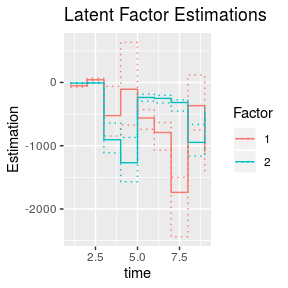# Overview

This document is part of the “DrBats” project whose goal is to implement exploratory statistical analysis on large sets of data with uncertainty. The idea is to visualize the results of the analysis in a way that explicitely illustrates the uncertainty in the data.

The “DrBats” project applies a Bayesian Latent Factor Model.

This project involves the following persons, listed in alphabetical order :

• Bénédicte Fontez (aut)

• Susan Holmes (aut)

• Gabrielle Weinrott (cre, aut)

# Bayesian Latent Factor Model

## Notations

• $$\mathbf{Y}$$ : observed response variable, $$y_1$$, …, $$y_i$$, …, $$y_N$$ are the rows each of length $$P$$

• $$\mathbf{W}$$ : is a low-rank matrix, with $$W_1$$, …, $$W_D$$ columns of length $$P$$, $$D \leq P$$

• $$\mathbf{\beta}$$ : a $$D \times N$$ matrix of factor loadings, and $$\beta_i$$ are the factor loadings of the $$i^{th}$$ individual observed

• $$\epsilon$$ : the $$P \times N$$ matrix of errors, with precision $$\sigma^2$$

We want to visualize $$\mathbf{Y}$$ in a lower dimension. The $$\mathbf{\beta}$$ are the coordinates of the observations in the lower dimension, $$\mathbf{W}$$ is the transition matrix, and $$\epsilon$$ represents the difference between the low-rank represenation and the actual data.

## Model

Without loss of generality, we assume that $$\mathbf{Y}$$ is centered. The Latent Factor Model is therefore:

$$\mathbf{Y_i}^T = \mathbf{W}\beta_i + \epsilon_i$$

For identifiability reasons, we will estimate a $$P \times D$$ positive lower triangular (PLT) matrix, instead of the full matrix $$\mathbf{W}$$. We can do a matrix decomposition of $$\mathbf{W}$$ as an orthogonal matrix and an upper-triangular matrix, $$\mathbf{W} = \mathbf{Q} \mathbf{R}$$. As such, we estimate the PLT matrix $$\mathbf{R}^T = \left(r_{j, k}\right)_{j = 1:P, k = 1:D}$$ with the rotation matrix $$\mathbf{Q}$$ known (for instance, estimated from the classical PCA):

$\mathbf{R}^T = \begin{pmatrix} r_{1, 1} & 0 & \cdots & 0\\ r_{2, 1} & r_{2, 2} & \ddots & 0 \\ r_{3, 1} & r_{3, 2} & \ddots & \vdots \\ \vdots & & \ddots & r_{P, D} \end{pmatrix}$

We assume for now that all rows of $$\mathbf{Y}$$ have the same variance, that is $$\sigma^2 \mathbf{1}_P$$. The full Bayesian model is:

$\begin{eqnarray}\label{model} \mathbf{Y_i}^T|\mathbf{R}^T, \beta_i, \sigma^2 &\overset{i.i.d.}{\sim}& \mathcal{N}_P(\mathbf{R}^T\beta_i, \sigma^2 \mathbf{1}_P) \end{eqnarray}$

$\begin{eqnarray*} \beta_i & \overset{i.i.d.}{\sim}& \mathcal{N}_D(0, \mathbf{1}_D) \\ r_{j, k} & \overset{i.i.d.}{\sim}& \mathcal{N}(0, \tau^2) \\ \sigma^2, \tau^2 &\sim& IG(0.001, 0.001) \end{eqnarray*}$

We assume that the non-null entries of the PLT matrix are independent, centered and normal, with same variance $$\tau^2$$.

To integrate information about uncertainty for interval-valued data, we can put an informative prior on the variance of each individual $$\mathbf{Y_i}$$. This could be in the form of a weight matrix, for instance $$\sigma^2 \Phi_i$$ where $$\Phi_i$$ is either fixed by the user, or estimated.

Finally, contrary to classical Principal Component Analysis, in this model the factor loadings $$\beta_i$$ for each individual are random variables. This allows for uncertainty of projection, resulting in non-elliptical confidence regions around the estimated factor loadings.

# Data Simulation

We can simulate data using $$\mathbf{Y_i}^T = \mathbf{R}^T\beta_i + \epsilon_i$$ with the function drbats.simul(). We choose a matrix $$\mathbf{R}$$, and then build $$\mathbf{Y_i}$$ by simulating $$\beta_i$$ and $$\epsilon_i$$ for each individual. To obtain the full matrix $$\mathbf{Y}$$, we stack the rows $$\mathbf{Y_i}$$.

The matrix $$\mathbf{R}$$ built in this package is, as previously stated, the result of the matrix decomposition of a full low-rank matrix $$\mathbf{W}$$. To choose an $$\mathbf{R}$$ that would resemble something out of an agronomic dataset, we build $$\mathbf{W}$$ to be the low-rank matrix of an extreme case of data found in agronomy: often times observations are signals that trend over time, with peaks at certain moments over the observation period. In addition, the number of observations can be small when these peaks occur.

To build $$\mathbf{W}$$ and subsequently, $$\mathbf{R}$$, we first simulate bi-modal signals observed unevenly over time.

suppressPackageStartupMessages(require(DrBats))
set.seed = 45
toydata <- drbats.simul(N = 5,
P = 150,
t.range = c(0, 1000),
b.range = c(0.2, 0.4),
c.range = c(0.6, 0.8),
b.sd = 5,
c.sd = 5,
y.range = c(-5, 5),
sigma2 = 0.2,
breaks = 8,
data.type = 'sparse.tend')
matplot(t(toydata$t), t(toydata$X), type = 'l', lty = 1, lwd = 1,
xlab = 'Time', ylab = ' ')
points(t(toydata$t), t(toydata$X), pch = '.')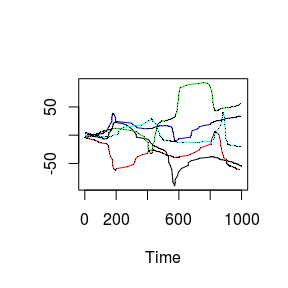For details please refer to the simul_and_project.pdf vignette.

We set the dimensionality of the low-rank matrix as the number of axes retained after Principal Component Analysis:

barplot(toydata$proj.pca$lambda.perc, ylim = c(0, 1),
col = mycol[1:length(toydata$proj.pca$lambda.perc)])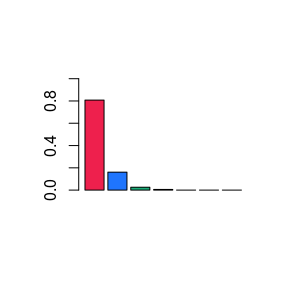##  "Number of retained axes: 2"

If you want to use the PCA rotation to anchor the latent factors, the function wlu() does an LU matrix decomposition of the matrix of latent factors.

# Model Inference

Now we fit a Stan model for the simulated data with a No-U-Turn Sampler using the modelFit() function, and the rstan package.

See the modelFit.pdf vignette for details.

fit <- modelFit(model = "PLT", var.prior = "IG", prog = "stan", Xhisto = toydata$Y.simul$Y,
nchains = 4, nthin = 50, niter = 10000, D = toydata$wlu$D)

The main.modelFit() function outputs an object called fit, containing the posterior estimates for the parameters of the model.

# Evaluation

For evaluation, we can convert the object to an mcmc.list to apply the diagnostic tests in the coda package manual. Our package also works on mcmc.lists for coherence.

We can plot the histogram of the posterior density of the data:

post <- postdens(codafit, Y = toydata$Y.simul$Y, D = toydata$wlu$D, chain = 1)
hist(post, main = "Histogram of the posterior density", xlab = "Density")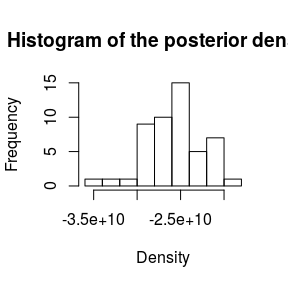It’s possible to visualize the projection of the observations onto the lower dimensional space with the function $$visbeta()$$. We can project onto the latent factors of our choice, here we chose the first and second (we didn’t have a choice actually since there are only two latent factors in the toy example). The uncertainty envelope at $$95 \%$$ is also plotted if we choose $$quant = c(0.05, 0.95)$$.

beta.res <- visbeta(codafit, toydata$Y.simul$Y, toydata$wlu$D, chain = 1, axes = c(1, 2), quant = c(0.05, 0.95))

ggplot2::ggplot() +
ggplot2::geom_path(data = beta.res$contour.df, ggplot2::aes(x = x, y = y, colour = ind)) + ggplot2::geom_point(data = beta.res$mean.df, ggplot2::aes(x = x, y = y, colour = ind)) +
ggplot2::ggtitle("Convex hull of Score Estimates")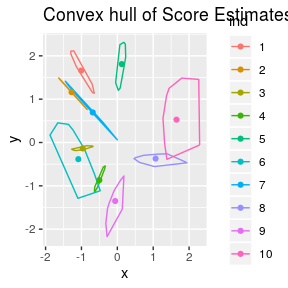W.res <- visW(codafit, toydata$Y.simul$Y, toydata$wlu$D, chain = 1, factors = c(1, 2))
W.df <- data.frame(time = 1:9, W.res\$res.W)
ggplot2::ggplot() +
ggplot2::geom_step(data = W.df, ggplot2::aes(x = time, y = Estimation, colour = Factor)) +
ggplot2::geom_step(data = W.df, ggplot2::aes(x = time, y = Lower.est, colour = Factor), linetype = 3) +
ggplot2::geom_step(data = W.df, ggplot2::aes(x = time, y = Upper.est, colour = Factor), linetype = 3) +
ggplot2::ggtitle("Latent Factor Estimations")